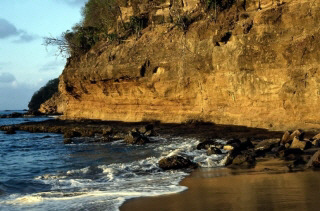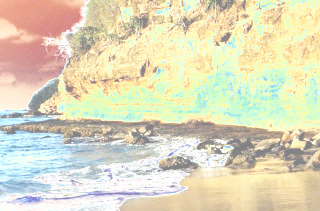﻿ MathematicalFunctionCommand Class | Leadtools.ImageProcessing.Color | Raster, Medical, Document Help
←Select platform
In This Topic ▼

# MathematicalFunctionCommand Class

Summary
Re-maps the image colors according to a mathematical function such as: square, logarithm, square root, sine and cosine.
Syntax
C#
Objective-C
C++/CLI
Java
Python
``public class MathematicalFunctionCommand : RasterCommand ``
``@interface LTMathematicalFunctionCommand : LTRasterCommand ``
````public class MathematicalFunctionCommand `
`    extends RasterCommand ````
``public ref class MathematicalFunctionCommand : public RasterCommand   ``
``class MathematicalFunctionCommand(RasterCommand): ``
Remarks
• This class uses the RGB color space.
• This class makes it possible to apply one of five mathematical functions to an image's pixel values: square, square root, logarithm, sine and cosine.
• Set the mathematical operation to be performed on each pixel's values in the Type property. The resulting values are multiplied by the Factor property value. The Factor property can be any value greater than 0. It is divided internally by different amounts, according to the type of the function being used.
• If the image has a region, the effect will be applied on the region only.
• This class supports 12 and 16-bit grayscale and 48 and 64-bit color images. Support for 12 and 16-bit grayscale and 48 and 64-bit color images is available only in the Document/Medical toolkits.
• This command does not support 32-bit grayscale images.

Mathematical Function - BeforeMathematical Function - AfterView additional platform support for this Mathematical function.

Example

Run the MathematicalFunctionCommand on an image with square function selected and factor = 50.

C#
````using Leadtools; `
`using Leadtools.Codecs; `
`using Leadtools.ImageProcessing.Color; `
` `
` `
`public void MathematicalFunctionCommandExample() `
`{ `
`   // Load an image `
`   RasterCodecs codecs = new RasterCodecs(); `
`   codecs.ThrowExceptionsOnInvalidImages = true; `
` `
`   RasterImage image = codecs.Load(Path.Combine(LEAD_VARS.ImagesDir, @"ImageProcessingDemo\NaturalFruits.jpg")); `
` `
`   // Prepare the command `
`   MathematicalFunctionCommand command = new MathematicalFunctionCommand(); `
`   command.Type = MathematicalFunctionCommandType.Square; `
`   command.Factor = 50; `
`   //Apply Square Function to this image. `
`   command.Run(image); `
`   codecs.Save(image, Path.Combine(LEAD_VARS.ImagesDir, "Result.jpg"), RasterImageFormat.Jpeg, 24); `
` `
`} `
` `
`static class LEAD_VARS `
`{ `
`   public const string ImagesDir = @"C:\LEADTOOLS22\Resources\Images"; `
`} ````
Requirements
Help Version 22.0.2023.3.31